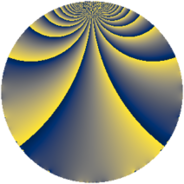Properties

 Label 297.1.hLevel $297$ Weight $1$ Character orbit 297.h Rep. character $\chi_{297}(10,\cdot)$ Character field $\Q(\zeta_{6})$ Dimension $2$ Newform subspaces $1$ Sturm bound $36$ Trace bound $0$

Related objects

Defining parameters

 Level: $$N$$ $$=$$ $$297 = 3^{3} \cdot 11$$ Weight: $$k$$ $$=$$ $$1$$ Character orbit: $$[\chi]$$ $$=$$ 297.h (of order $$6$$ and degree $$2$$) Character conductor: $$\operatorname{cond}(\chi)$$ $$=$$ $$99$$ Character field: $$\Q(\zeta_{6})$$ Newform subspaces: $$1$$ Sturm bound: $$36$$ Trace bound: $$0$$

Dimensions

The following table gives the dimensions of various subspaces of $$M_{1}(297, [\chi])$$.

Total New Old
Modular forms 18 6 12
Cusp forms 6 2 4
Eisenstein series 12 4 8

The following table gives the dimensions of subspaces with specified projective image type.

$$D_n$$ $$A_4$$ $$S_4$$ $$A_5$$
Dimension 2 0 0 0

Trace form

 $$2 q - q^{4} - q^{5} + O(q^{10})$$ $$2 q - q^{4} - q^{5} + q^{11} - q^{16} - q^{20} + 2 q^{23} + q^{31} - 2 q^{37} - 2 q^{44} - q^{47} - q^{49} + 2 q^{53} - 2 q^{55} - q^{59} + 2 q^{64} + q^{67} + 2 q^{71} + 2 q^{80} - 4 q^{89} + 2 q^{92} + q^{97} + O(q^{100})$$

Decomposition of $$S_{1}^{\mathrm{new}}(297, [\chi])$$ into newform subspaces

Label Dim. $$A$$ Field Image CM RM Traces $q$-expansion
$a_{2}$ $a_{3}$ $a_{5}$ $a_{7}$
297.1.h.a $2$ $0.148$ $$\Q(\sqrt{-3})$$ $D_{3}$ $$\Q(\sqrt{-11})$$ None $$0$$ $$0$$ $$-1$$ $$0$$ $$q+\zeta_{6}^{2}q^{4}+\zeta_{6}^{2}q^{5}+\zeta_{6}q^{11}-\zeta_{6}q^{16}+\cdots$$

Decomposition of $$S_{1}^{\mathrm{old}}(297, [\chi])$$ into lower level spaces

$$S_{1}^{\mathrm{old}}(297, [\chi]) \cong$$ $$S_{1}^{\mathrm{new}}(99, [\chi])$$$$^{\oplus 2}$$